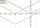Roots

Determine the quadratic equation absolute coefficient q, that the equation has a real double root and the root x calculate:Result

q =  4.05
x =  -0.9

Solution:Leave us a comment of example and its solution (i.e. if it is still somewhat unclear...):Be the first to comment!To solve this example are needed these knowledge from mathematics:

Looking for help with calculating roots of a quadratic equation?

Next similar examples:Quadratic equation ? has roots x1 = 80 and x2 = 78. Calculate the coefficients b and c.Find the roots of the quadratic equation: 3x2-4x + (-4) = 0.
3. EquationEquation ? has one root x1 = 8. Determine the coefficient b and the second root x2.
4. DiscriminantDetermine the discriminant of the equation: ?
5. Variations 4/2Determine the number of items when the count of variations of fourth class without repeating is 600 times larger than the count of variations of second class without repetition.
6. Equation with abs valueHow many solutions has the equation ? in the real numbers?
7. CombinationsFrom how many elements we can create 990 combinations 2nd class without repeating?
8. Solve 3Solve quadratic equation: (6n+1) (4n-1) = 3n2Which of the points belong function f:y= 2x2- 3x + 1 : A(-2, 15) B (3,10) C (1,4)
10. CombinationsHow many elements can form six times more combinations fourth class than combination of the second class?
11. AlgebraX+y=5, find xy (find the product of x and y if x+y = 5)Solve quadratic equation: 2x2-58x+396=0If k(x+6)= 4x2 + 20, what is k(10)=?Find variable P: PP plus P x P plus P = 160Solve two equations with two unknowns: 400x+120y=147.2 350x+200y=144We want to prove the sentence: If the natural number n is divisible by six, then n is divisible by three. From what assumption we started?There is geometric sequence with a1=5.7 and quotient q=-2.5. Calculate a17.